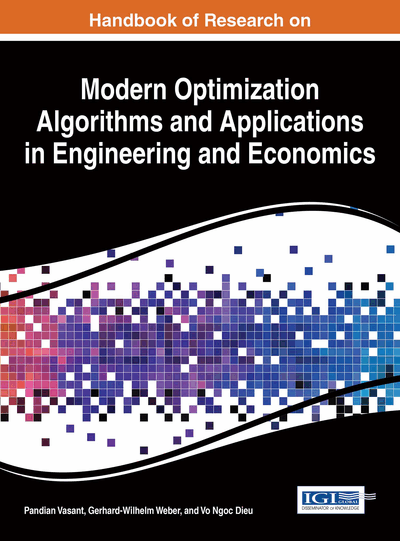# Swarm Intelligence for Multiobjective Optimization of Extraction Process

T. Ganesan (The University of Adelaide, Australia), I. Elamvazuthi (Universiti Technologi PETRONAS, Malaysia) and P. Vasant (Universiti Technologi PETRONAS, Malaysia)
DOI: 10.4018/978-1-4666-9644-0.ch020

## Abstract

Multi objective (MO) optimization is an emerging field which is increasingly being implemented in many industries globally. In this work, the MO optimization of the extraction process of bioactive compounds from the Gardenia Jasminoides Ellis fruit was solved. Three swarm-based algorithms have been applied in conjunction with normal-boundary intersection (NBI) method to solve this MO problem. The gravitational search algorithm (GSA) and the particle swarm optimization (PSO) technique were implemented in this work. In addition, a novel Hopfield-enhanced particle swarm optimization was developed and applied to the extraction problem. By measuring the levels of dominance, the optimality of the approximate Pareto frontiers produced by all the algorithms were gauged and compared. Besides, by measuring the levels of convergence of the frontier, some understanding regarding the structure of the objective space in terms of its relation to the level of frontier dominance is uncovered. Detail comparative studies were conducted on all the algorithms employed and developed in this work.
Chapter Preview
Top

## Introduction

Multi-criteria or multi-objective (MO) scenarios have become increasingly prevalent in industrial engineering environments (Statnikov & Matusov, 1995; Zhang and Li, 2007; Li and Zhou, 2011). MO optimization problems are commonly tackled using the concept of Pareto-optimality to trace-out the non-dominated solution options at the Pareto curve (Zitzler & Thiele, 1998; Deb et al., 2002). Other methods include the weighted techniques which involve objective function aggregation resulting in a master weighted function. This master weighted function is then solved for various weight values (which are usually fractional) (Fishburn, 1967; Triantaphyllou, 2000; Luyben. & Floudas, 1994; Das & Dennis, 1998). Using these techniques, the weights are used to consign relative importance or priority to the objectives in the master aggregate function. Hence, alternative near-optimal solution options are generated for various values of the scalars. In this chapter, the Normal Boundary Intersection (NBI) scheme (Das & Dennis, 1998) was used as a scalarization tool to construct the Pareto frontier. In Sandgren (1994) and Statnikov & Matusov (1995), detail examples and analyses on MO techniques for problems in engineering optimization are presented.

Many optimization techniques have been implemented for solving the extraction process problem (e.g. Hismath et al., 2011; Jie and Wei, 2008). In addition, evolutionary techniques such as DE have also been employed for extraction process optimization (Ubaidullah et al., 2012). The MO problem considered in this work was formulated by Shashi et al, (2010). This problem involves the optimization of the yields of certain chemical products which are extracted from the Gardenia Jasminoides Ellis fruit. The MO optimization model was developed in Shashi et al, (2010) to maximize the extraction yields which are the three bioactive compounds; crocin, geniposide and total phenolic compounds. The optimal extraction parameters which construct the most dominant Pareto frontier are then identified such that the process constraints remain unviolated. In Shashi et al., (2010), the MO problem was tackled using the real-coded Genetic Algorithm (GA) approach to obtain a single individual optima and not a Pareto frontier. In that work, measurement metrics were not employed to evaluate the solution quality in detail. In addition, the work done in Shashi et al., (2010) focused on modeling the system rather than optimizing it. The authors of that work employed only one optimization technique and did not carry out extensive comparative analysis on the optimization capabilities. Due to this setbacks, these factors are systematically addressed in this chapter to provide some insights on the optimization of the extraction process.

## Key Terms in this Chapter

Multiobjective Optimization: Optimization problems which are represented with more than one objective functions.

Gravitational Search Algorithms (GSA): A type of metaheuristic algorithm integrates ideas from Newtonian gravitational laws to search for optimal solutions in the objective space.

Gardenia Jasminoides Ellis: A fruit that produces chemical products such as crocins, geniposide and the phenolic compounds which are widely used in the food industry as a natural food colorants (dyes). These compounds also have high antioxidant capabilities which make this fruit valuable for medicinal uses.

Particle Swarm Optimization (PSO): A type of metaheuristic algorithm that uses concepts from swarming behavior of organisms to search for optimal solutions.

Metaheuristics: A framework consisting of a class of algorithms employed to find good solutions to optimization problems by iterative improvement of solution quality.

Measurement Metrics: Mathematical metrics used to identify and measure solution characteristics such as; degree of convergence, diversity and dominance.

Hopfield-Enhanced Particle Swarm Optimization (HoPSO): A novel swarm intelligence technique which integrates concepts from the Hopfield Neural Nets and swarming behavior of organisms to improve the solution dominance of the standard PSO when solving multiobjective problems.

Normal Boundary Intersection: A solution method where a multiobjective optimization problem is geometrically reduced to a beta-sub problem and then solved as a weighted single objective optimization problem.

## Complete Chapter List

Search this Book:
Reset